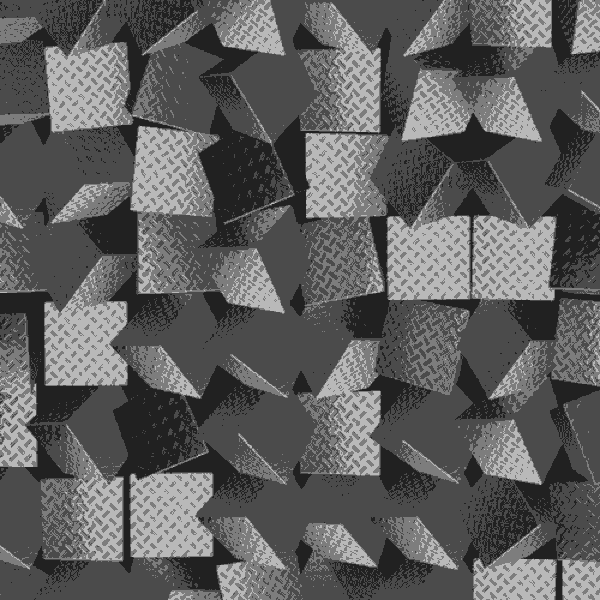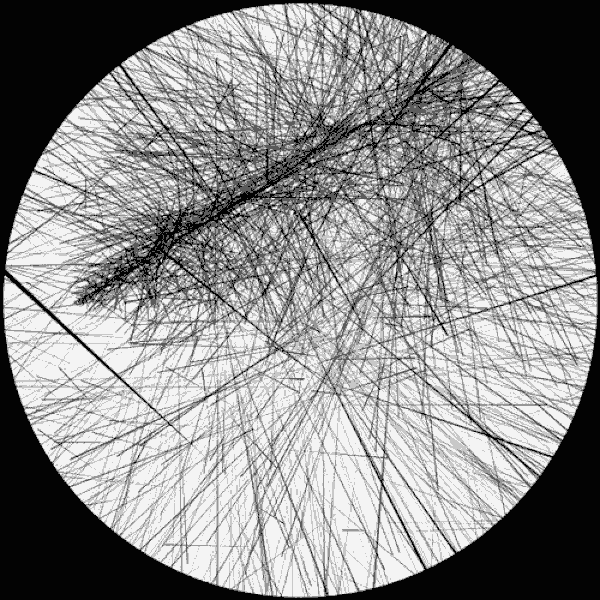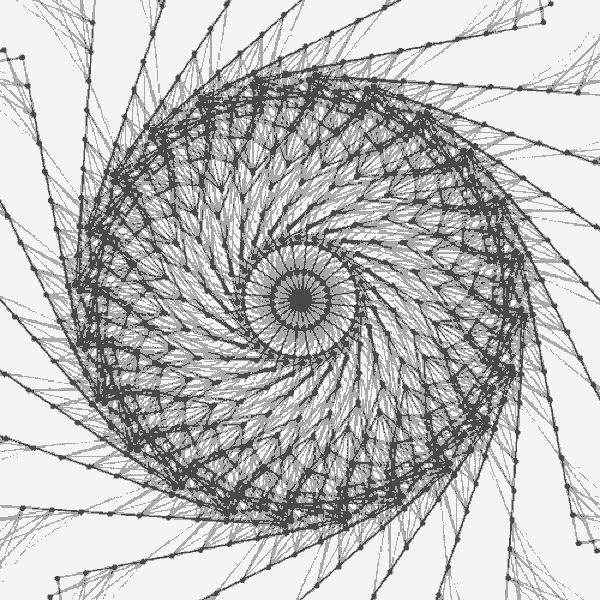Feedback

# Mind Blowing Calculation Tips For Binary Number System!

News
> Mind Blowing Calculation Tips For Binary Number System!

The binary number system is used widely in programming and coding. To some people, this might seem too difficult or impossible to grasp or implement, but it has an easy, elegant logic behind it.

Are you ready to get your world rocked and double your horizon?

## It is pretty easy to multiply or divide and multiply a number by two in the binary system: you shift all numbers to left to multiply and to left to divide them.For example: 1938 : 11110010010.969 : 01111001001.484 : 00111100100 (carry over the 1).242 : 00011110010.121 : 00001111001..60 : 00000111100..30 : 00000011110..15 : 00000001111...7 : 00000000111 ...3 : 000000000111 : 1We actually do the same thing in a decimal system while dividing a number by 10. We shift the numbers one by one and get rid of the zero at the end.

## This tip is used often in animations after it started out with computers.The 'pictures' you see on your screen consist of what we call 'pixel.' Now that we are on the subject, the word 'pixel' is made up of 'picture and element.'Let's look at the upper case 'A' letter:00000000 = 000111100 = 6001000010 = 6601000010 = 6601111110 = 12601000010 = 6601000010 = 6600000000 = 0 These are the numbers coded in a way that they give you the letter 'a' on your screen.

## At this point, if you multiply all numbers by two, the pixels making up the letter 'a' will shift to left, and right if you divide them by two.That's how you use math to shift images on your screen.Even in the very simple games consisting of a stick-man walking from left to right side of the screen on our desktops with 8-bit processors, people used to code using such numeric functions.Nothing is as easy as it looks.
REACT TO THIS CONTENT WITH EMOJI!
1
1
1
1
1
0
0
WHAT ARE ONEDIO MEMBERS SAYING?